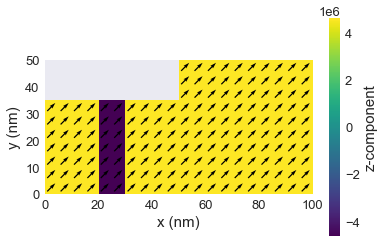# Getting started 01: Geometry and magnetisation¶

Interactive online tutorial:In this tutorial we explore how geometries and magnetisation states can be specified. The package we use to define finite difference meshes and fields is called discretisedfield and we have to import it before we start.

In :
import discretisedfield as df
import micromagneticmodel as mm

# The following line enables plotting inside the notebook.
%matplotlib inline


## Mesh¶

Let us say that we need to define a nanocube mesh with edge length $L=100\,\text{nm}$ and discretisation cell $(d, d, d)$, with $d=10 \,\text{nm}$. For that we need to define two points $p_{1}$ and $p_{2}$ between which the mesh spans and pass them (together with the discretisation cell) to the Mesh class:

In :
L = 100e-9  # edge length (m)
d = 10e-9  # cell size (m)

p1 = (0, 0, 0)  # first point of the cuboid containing simulation geometry
p2 = (L, L, L)  # second point
cell = (d, d, d)  # discretisation cell

region = df.Region(p1=p1, p2=p2)
mesh = df.Mesh(region=region, cell=cell)  # mesh definition


We can then inspect some basic parameters of the mesh:

### Edge length:¶

In :
mesh.region.edges  # edge length

Out:
(1e-07, 1e-07, 1e-07)

### Number of discretisation cells in all three directions:¶

In :
mesh.n  # number of cells

Out:
(10, 10, 10)

### Minimum mesh domain coordinate:¶

In :
mesh.region.pmin  # minimum mesh domain coordinate

Out:
(0.0, 0.0, 0.0)

### Maximum mesh domain coordinate:¶

In :
mesh.region.pmax  # maximum mesh domain coordinate

Out:
(1e-07, 1e-07, 1e-07)

### Visualise the mesh domain and a discretisation cell:¶

Using k3d interactive plots:

In :
mesh.k3d()


Using matplotlib

In :
mesh.mpl()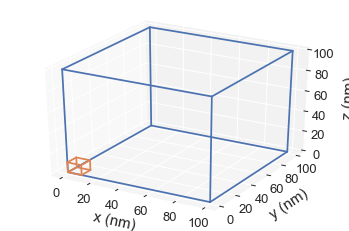## Field¶

After we defined a mesh, we can define different finite difference fields. For that, we use Field class. We need to provide:

1. Mesh,
2. Dimension of the data values,
3. Value of the field.

Let us define a 3D-vector field (dim=3) that is uniform in the $(1, 0, 0)$ direction.

In :
m = df.Field(mesh, dim=3, value=(1, 0, 0))


A simple slice visualisation of the mesh in the $z$ direction at $L/2$ is:

In :
m.plane('z').mpl()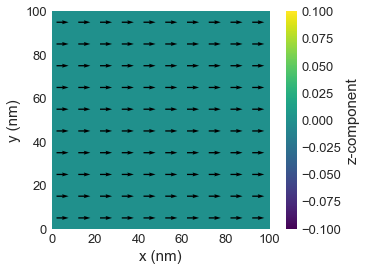Similarly, a three-dimensional interactive visualisation is:

In :
m.plane('z').k3d_vector(head_size=30)


### Spatially varying field¶

When we defined a uniform vector field, we used a tuple (1, 0, 0) to define its value. However, we can also provide a Python function if we want to define a non-uniform field. This function takes the position in the mesh as input, and returns a value that the field should have at that point:

In :
def m_value(pos):
x, y, z = pos  # unpack position into individual components
if x > L/5:
return (1, 0, 0)
else:
return (-1, 1, 0)

m = df.Field(mesh, dim=3, value=m_value)

m.plane('z').mpl()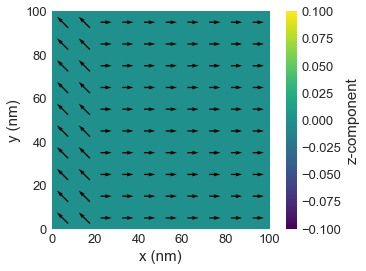The field object can be treated as a function - if we pass a position tuple to the function, it will return the vector value of the field at that location:

In :
point = (0, 0, 0)
m(point)

Out:
(-1.0, 1.0, 0.0)

In micromagnetics, the saturation magnetisation $M_\mathrm{s}$ is typically constant (at least for each position). The Field constructor accepts an additional parameter norm which we can use for that:

In :
Ms = 8e6  # saturation magnetisation (A/m)
m = df.Field(mesh, dim=3, value=m_value, norm=Ms)

m((50e-9, 0, 0))

Out:
(8000000.0, 0.0, 0.0)

### Spatially varying $M_\mathrm{s}$¶

By defining different norms, we can specify different geometries, so that $M_\text{s}=0$ outside the mesh. For instance, let us assume we want to define a sphere of radius $L/2$ and magnetise it in the negative $y$ direction.

In :
mesh = df.Mesh(p1=(-L/2, -L/2, -L/2), p2=(L/2, L/2, L/2), cell=(d, d, d))

def Ms_value(pos):
x, y, z = pos
if (x**2 + y**2 + z**2)**0.5 < L/2:
return Ms
else:
return 0

m = df.Field(mesh, dim=3, value=(0, -1, 0), norm=Ms_value)

m.plane('z').mpl()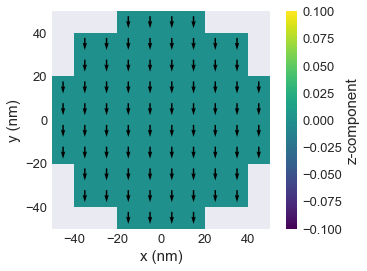Similarly, we can inspect the defined domain using k3d and inspecting the field's norm.

In :
m.norm.k3d_nonzero()


## Exercise 1¶

a) The code in the next cell defines a thin film of thickness $t$ in the $xy$ plane. Extend it to define the disk geometry of thickness $t = 10 \,\text{nm}$ and diameter $d = 120 \,\text{nm}$. The saturation magnetisation $M_\mathrm{s} = 10^7\mathrm{A/m}$. The disk is centred around the origin (0, 0, 0). The magnetisation is $\mathbf{m} = (1, 0, 0)$ at all points.

In :
t = 10e-9  # thickness (m)
d = 120e-9  # diameter (m)
cell = (5e-9, 5e-9, 5e-9)  # discretisation cell size (m)
Ms = 1e7  # saturation magnetisation (A/m)

region = df.Region(p1=(-d/2, -d/2, -t/2), p2=(d/2, d/2, t/2))
mesh = df.Mesh(region=region, cell=cell)

def Ms_value(pos):
x, y, z = pos
# insert missing code here
if (x**2 + y**2)**0.5 < d/2:
return Ms
else:
return 0
# return Ms

m = df.Field(mesh, dim=3, value=(1, 0, 0), norm=Ms_value)



b) Extend the previous example in the next cell so that the magnetisation is:

$$\mathbf{m} = \begin{cases} (-1, 0, 0) & \text{for } y \le 0 \\ (1, 0, 0) & \text{for } y > 0 \end{cases}$$

with saturation magnetisation $10^{7} \,\text{A}\,\text{m}^{-1}$.

In :
t = 10e-9  # thickness (m)
d = 120e-9  # diameter (m)
cell = (5e-9, 5e-9, 5e-9)  # discretisation cell size (m)
Ms = 1e7  # saturation magnetisation (A/m)

region = df.Region(p1=(-d/2, -d/2, -t/2), p2=(d/2, d/2, t/2))
mesh = df.Mesh(region=region, cell=cell)

def Ms_value(pos):
x, y, z = pos
# Copy code from exercise 1a.
if (x**2 + y**2)**0.5 < d/2:
return Ms
else:
return 0
# return Ms

def m_value(pos):
x, y, z = pos
# Insert missing code here to get the right magnetisation.
if y <= 0:
return (-1, 0, 0)
else:
return (1, 0, 0)
# return (1, 0, 0)

m = df.Field(mesh, dim=3, value=m_value, norm=Ms_value)



## Exercise 2¶

Extend the code in the following cell to define the following geometry with $10\,\text{nm}$ thickness:The magnetisation saturation is $8 \times 10^{6} \,\text{A}\,\text{m}^{-1}$ and the magnetisation direction is as shown in the figure.

In :
cell = (5e-9, 5e-9, 5e-9)  # discretisation cell size (m)
Ms = 8e6  # saturation magnetisation (A/m)

region = df.Region(p1=(0, 0, 0), p2=(100e-9, 50e-9, 10e-9))
mesh = df.Mesh(region=region, cell=cell)

def Ms_value(pos):
x, y, z = pos
# Insert missing code here to get the right shape of geometry.
if x < 50e-9 and y > 35e-9:
return 0
else:
return Ms
# return Ms

def m_value(pos):
x, y, z = pos
if 20e-9 < x <= 30e-9:
return (1, 1, -1)
else:
return (1, 1, 1)

m = df.Field(mesh, dim=3, value=m_value, norm=Ms_value)

m.plane('z').mpl()